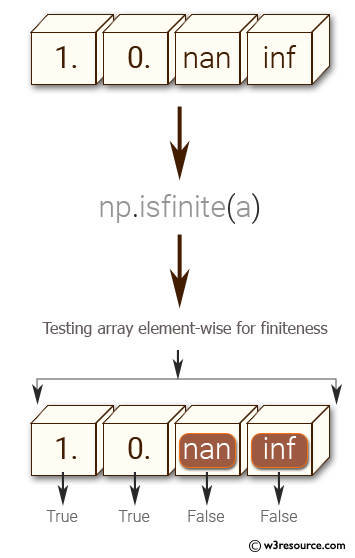﻿ NumPy: Test a given array element-wise for finiteness (not infinity or not a Number) - w3resource

# NumPy: Test a given array element-wise for finiteness (not infinity or not a Number)

## NumPy: Basic Exercise-5 with Solution

Write a NumPy program to test a given array element-wise for finiteness (not infinity or not a Number).

Sample Solution :

Python Code :

``````import numpy as np
a = np.array([1, 0, np.nan, np.inf])
print("Original array")
print(a)
print("Test a given array element-wise for finiteness :")
print(np.isfinite(a))
``````

Sample Output:

```Original array
[  1.   0.  nan  inf]
Test a given array element-wise for finiteness :
[ True  True False False]
```

Pictorial Presentation:Python Code Editor:

Have another way to solve this solution? Contribute your code (and comments) through Disqus.

What is the difficulty level of this exercise?

﻿

Inviting useful, relevant, well-written and unique guest posts